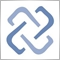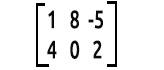# Discussion of article "Data Science and Machine Learning part 03: Matrix Regressions"Moderator
249267

New article Data Science and Machine Learning part 03: Matrix Regressions has been published:

This time our models are being made by matrices, which allows flexibility while it allows us to make powerful models that can handle not only five independent variables but also many variables as long as we stay within the calculations limits of a computer, this article is going to be an interesting read, that's for sure.

If you paid attention to the previous two articles you'll notice the big issue I had is programming models that could handle more independent variables, by this I mean dynamically handle more inputs because when it comes to creating strategies we are going to deal with hundreds of data, so we want to be sure that our models can cope with this demand### Matrix

for those that skipped mathematics classes, a matrix is a rectangular array or table of numbers or other mathematical objects arranged in rows and columns which is used to represent a mathematical object or a property of such an object

for exampleelephant in the room,

the way we read the matrices is rows x columns the above matrix is a 2x3 matrix meaning 2 rows, 3 columns

It is no doubt that matrices play a huge part in how modern computers process information and compute large numbers, the main reason why they are able to achieve such a thing is because data in matrix is stored in the array form that computers can read and manipulate. So let's see their application in machine learning,

Author: Omega J Msigwa